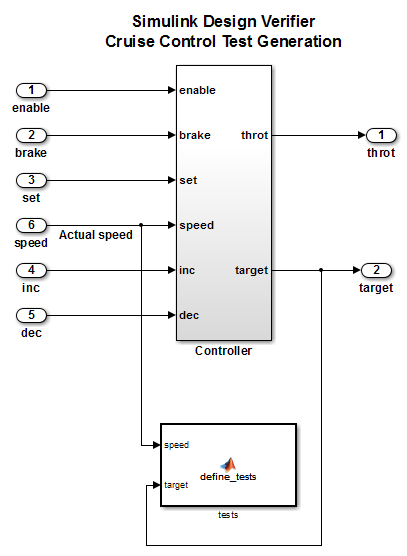# sldv.condition

Test condition function for Stateflow charts and MATLAB Function blocks

## Syntax

``sldv.condition(expr)``

## Description

example

````sldv.condition(expr)` specifies that `expr` is true for every time step in a generated test case. Use any valid Boolean expression for `expr`.```

This function has no output and no impact on its parenting function, other than any indirect side effects of evaluating `expr`. If you issue this function from the MATLAB® command line, the function has no effect.

Intersperse `sldv.condition` test conditions within MATLAB code or separate the conditions into a verification script.

The Test conditions option in the Test generation pane applies to test conditions represented with the `sldv.condition` function and with the Test Condition block.

## Examples

collapse all

Add a test objective and test conditions by using the MATLAB Function block.

Open the `sldvdemo_cruise_control` model and save it as `ex_sldvdemo_cruise_control`.

Remove the Test Condition block for the `speed` block signal. Instead of the Test Condition block, this example uses `sldv.test` and `sldv.condition`.

From the User-Defined Functions library, add a MATLAB Function block:

1. Name the block `tests`.

2. Open the block and add this code:

```function define_tests(speed, target) %#codegen sldv.condition(speed >= 0 && speed <= 100); sldv.test(speed > 60 && target > 40 && target < 50); sldv.test(speed < 20 && target > 50);```
3. In the Editor tab, click and close the editor.

4. Connect the block to the signals for the `speed` block and for the `target` block.

Save the changes to the `ex_environment_controller` model.To generate test cases, on the Design Verifier tab, click .

## Input Arguments

collapse all

MATLAB expression, for example, `x > 0`.

## Alternatives

Instead of using the `sldv.condition` function, you can insert a Test Condition block in your model. Using `sldv.condition` instead of a Test Condition block offers several benefits, described in What is Test Case Generation?.

You can also specify test conditions by using MATLAB for code generation without using the `sldv.condition` function. Using `sldv.condition` instead of directly using MATLAB for code generation eliminates the need to:

• Express the constraints with Simulink® blocks.

• Explicitly connect the condition output to a Simulink block.